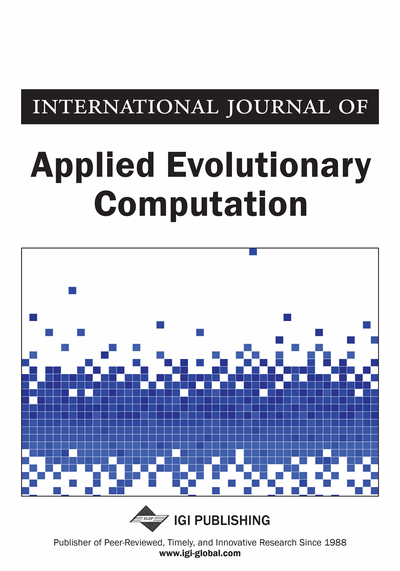# Calibration of the Parameters of a Model of an Engineering System Using the Global Optimization Method

Marwa Elhajj (University of Versailles, Versailles, France), Rafic Younes (Lebanese University, Beirut, Lebanon), Sebastien Charles (University of Versailles, Versailles, France) and Eric Padiolleau (French Mechanical Technical Center, Paris, France)
DOI: 10.4018/ijaec.2014070102
Available
\$37.50
No Current Special Offers

## Abstract

The calibration of the model is one of the most important steps in the development of models of engineering systems. A new approach is presented in this study to calibrate a complex multi-domain system. This approach respects the real characteristics of the circuit, the accuracy of the results, and minimizes the cost of the experimental phase. This paper proposes a complete method, the Global Optimization Method for Parameter Calibration (GOMPC). This method uses an optimization technique coupled with the simulated model on simulation software. In this paper, two optimization techniques, the Genetic Algorithm (GA) and the two-level Genetic Algorithm, are applied and then compared on two case studies: a theoretical and a real hydro-electromechanical circuit. In order to optimize the number of measured outputs, a sensitivity analysis is used to identify the objective function (OBJ) of the two studied optimization techniques. Finally, results concluded that applying GOMPC by combining the two-level GA with the simulated model was an efficient solution as it proves its accuracy and efficiency with less computation time. It is believed that this approach is able to converge to the expected results and to find the system's unknown parameters faster and with more accuracy than GA.
Article Preview
Top

## 1. Introduction

Many engineering system models rely on several unknown parameters. Their values need to be identified by direct or indirect measurements. However, in many cases, this is not possible (Wang, 1997). In the case of hydro-electromechanical systems treated here, some of the unknown parameters vary with time and the system consumption (efficiency, size of the cylinder, etc…). Other parameters, given in the technical documentation of a component, represent the values ​​when the component works separately. Therefore, they do not reflect the actual operating conditions of the system, the interactions between the components and their influence during operation. For these reasons, the identification of the system’s real values becomes a necessity, hence the importance of calibrating the studied system. The calibration is defined as the identification of the model's unknown parameters and/or structure based on measurements, and prior knowledge (Dai & al., 2009). This technique allows us to estimate the model parameters that cannot be measured. This is achieved by comparing the model results with measurements and adjusting the parameters of the model to obtain a match between the model's predictions and the experimental results (Dai & al., 2009). The purpose of the calibration is ultimately to find the values of the unknown parameters, which minimize the deviation between the simulated and experimental values.

These unknown parameters are calibrated by solving a constrained optimization problem for which an OBJ is to be minimized or maximized subject to the constraints. In our case, the OBJ is defined as the sum of the squared differences between the simulated and experimental outputs. This function should be minimized so that the simulation outputs converge to the experimental ones. The OBJ can be expressed as follows (1):OBJ =*) (1) where s is the total number of evaluated simulation outputs, n is the total count of simulation steps,is the simulated value of the kth measured simulation physical output at the ith simulation step andis the experimental value of the kth measured physical output at the ith simulation step.

This OBJ is subject to two constraints:

where Oi is the ith calibrated parameter, Oi min and Oi max are the lower and upper bounds respectively of Oi.where Xk is the kth physical output, Oi is the ith calibrated parameter and t is the time.

## Complete Article List

Search this Journal:
Reset
Open Access Articles: Forthcoming
Volume 13: 4 Issues (2022): 1 Released, 3 Forthcoming
Volume 12: 4 Issues (2021)
Volume 11: 4 Issues (2020)
Volume 10: 4 Issues (2019)
Volume 9: 4 Issues (2018)
Volume 8: 4 Issues (2017)
Volume 7: 4 Issues (2016)
Volume 6: 4 Issues (2015)
Volume 5: 4 Issues (2014)
Volume 4: 4 Issues (2013)
Volume 3: 4 Issues (2012)
Volume 2: 4 Issues (2011)
Volume 1: 4 Issues (2010)
View Complete Journal Contents Listing